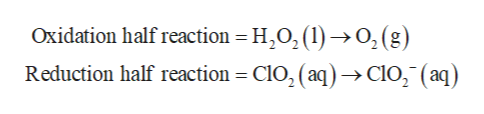# Balance the following redox reaction if it occurs in basic solution. What are the coefficients in front of ClO2 and H2O in the balanced reaction? H2O2(l) + ClO2(aq) → ClO2(aq) + O2(g)

Question
32 views
Balance the following redox reaction if it occurs in basic solution. What are the coefficients in front of ClOand H2O in the balanced reaction?

H2O2(l) + ClO2(aq) → ClO2(aq) + O2(g)

check_circle

Step 1

The given reaction is shown below.

Step 2

The decrease in oxidation number is called reduction reaction while the increase in the oxidation number is called oxidation reaction.

The first step for balancing the redox reaction is to divide the given reaction into two half reactions as shown below.help_outlineImage TranscriptioncloseOxidation half reaction = H2O2(1) >O,(g) Reduction half reaction = ClO2 ( aq)->ClO2 (aq) fullscreen
Step 3

In oxidation half reaction, the oxidation state of Oxygen changes from -1 to 0, whereas in reduction half reaction, the oxidation state of Oxygen changes from +4 to +3.

The next step involves balancing of atoms other than Oxy...

### Want to see the full answer?

See Solution

#### Want to see this answer and more?

Solutions are written by subject experts who are available 24/7. Questions are typically answered within 1 hour.*

See Solution
*Response times may vary by subject and question.
Tagged in

### Redox Reactions